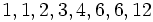# Landau's function

## Definition

Letbe a nonnegative integer. The Landau's function ofis defined in the following equivalent ways:

• The maximum possible value for the least common multiple of the parts in an unordered integer partition of.
• The maximum of the orders of all elements in the symmetric group of degree.

The value atis defined to be.

## Behavior

The ID of the sequence in the Online Encyclopedia of Integer Sequences is A000793

## =Initial values

The values of Landau's function foris.

## Relation with other functions

• lcm of all numbers so far is the exponent of the symmetric group of degree.
• factorial is the order (i.e., cardinality) of the symmetric group of degree.